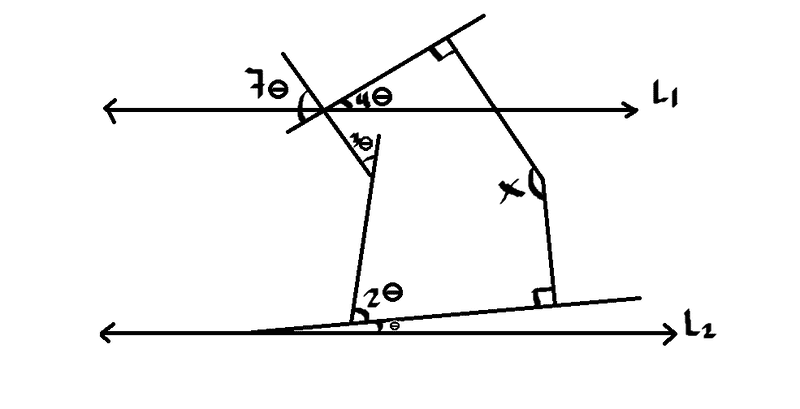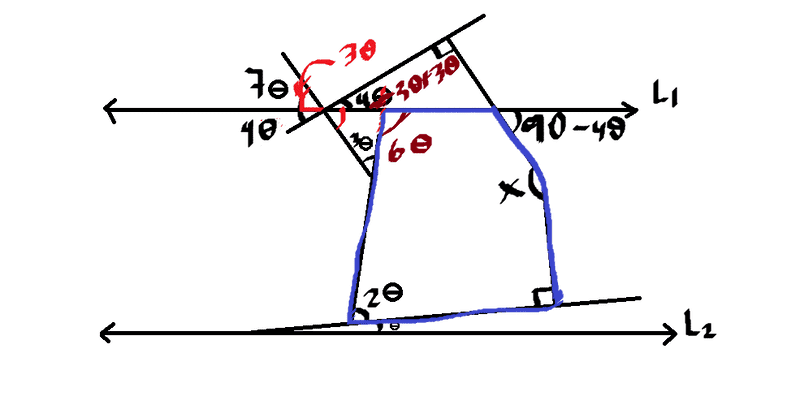# Tips for this geometry problem please

david3305

## Homework Statement

Find x. L1 and L2 are parallelChoices:
a)100
b)120
c)140
d)150
e)135

## Homework EquationsFrom the image, the angles of the polygon in blue should satisfy:
6θ + 90 + 4θ + 2θ + 90 + x = 540
12θ + x = 360
x = 360 - 12θ

## The Attempt at a Solution

I couldn't figure out how to advance from there so I resorted to a dirty trick using the values from the choices.
Noticing that every value of each choice is a multiply of 5 then the value of θ in the equation must be:
θ = 5n
So it follows that:
x = 360-12(5n)
Then by trial and error:
x = 180 for n = 3
x = 120 for n = 4
x = 60 for n = 5
From the image x should be obtuse so then I conclude that x must be equal to 120
and the answer would be the letter 'b'. I know I got it right cause my book says that's the correct answer. However since this is a section of my book were no development of the solution is presented (just the final answer) I'm asking for some tips on other methods to solve this. Perhaps I'm overlooking something...

#### Attachments

Gold Member
I found it most efficacious to solve for ##\theta## first. Note that from the lower left moving rightward on L2 you can follow the turns until you're moving leftward along L1 and you will have turned ##180^\circ## by a sequence of angles ##\theta, 2\theta, 3\theta, (7-4)\theta##. So with that you can solve for ##\theta##.

I also note that the drawing is very much not to scale w.r.t. angles.

•david3305
david3305

I'm interested to see another way of solving for X that doesn't involve using the polygon like I did.

And yes, the drawing is not to scale at all.

LowPostCount
If the lower θ and upper 4θ were both zero, then X would be 180°, so the answer is whatever the upper one is less the lower one, subtracted from 180°. So without solving for θ (which jambaugh already did) it looks to me like X = 180° - (4θ - θ), or 180° - 3θ.

Does that help?

david3305
yeah tbh I was kinda lazy to think about it, just following jambaugh's way I can find the following is true:

(θ) + (90) + (180 - x) + (90 - 4θ) = 180
-3θ + 360 - x = 180
180 - 3θ = x

By the same reasoning we already know:
θ + 2θ + 3θ + 3θ = 180
9θ = 180
θ = 20
so:
180 - 3(20) = x
x = 120